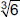# User Forum

Subject :IMO    Class : Class 6

Subject :IMO    Class : Class 6

## Ans 1:

Class : Class 1

Subject :IMO    Class : Class 5

Class : Class 7

Class : Class 8

Class : Class 3
1/6of 240=40

## Ans 4:

Class : Class 4
ANSWER IS B) 1/6 2/3 OF 240=160 1/6 OF 240=40 160 + 40= 200 240 - 200=40 40 IS 1/6 OF 240 AS 240/6=40 SO,1/6 OF THE ANIMALS IN THE FARM ARE TURKEYS

## Ans 5:

Class : Class 2
it can be both b and c

Subject :IMO    Class : Class 3

## Ans 1:

Class : Class 4

Class : Class 1

There are 6 triangles and 13 circles. So altogether there are 19 circles and triangles altogether in the given figure.

## Ans 3:

Class : Class 4

Subject :IMO    Class : Class 6

## Ans 1:

Class : Class 7
J=12

Subject :IMO    Class : Class 6

## Ans 1:

Class : Class 7
1/2 is the fraction taken by each

Subject :IMO    Class : Class 4

Class : Class 1

Subject :IMO    Class : Class 8

## Ans 1:

Class : Class 9
0 is both a number and the numerical digit used to represent that number in numerals. The number 0 fulfills a central role in mathematics as the additive identity of the integers, real numbers, and many other algebraic structures. As a digit, 0 is used as a placeholder in place value systems.

Subject :IMO    Class : Class 8

## Ans 1:

Class : Class 9
0 is both a number and the numerical digit used to represent that number in numerals. The number 0 fulfills a central role in mathematics as the additive identity of the integers, real numbers, and many other algebraic structures. As a digit, 0 is used as a placeholder in place value systems.

Subject :IMO    Class : Class 8

Class : Class 1

We have,